ggplot2 offers the fantastic option for displaying complex data in forms of 'many multiple', i.e. the facets. From the example of facet_grid:

p <- ggplot(mpg, aes(displ, cty)) + geom_point()
p + facet_grid(drv ~ cyl) + theme_bw()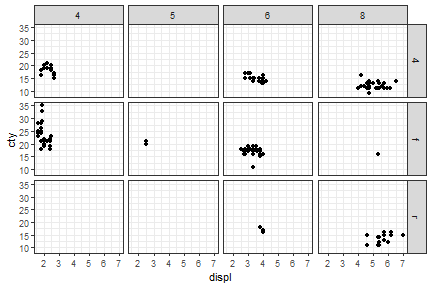In the above example, the panel's borders are drawn with the default settings of theme_bw().

If we desire the the axis lines, such as those given in this package, the distinction of the panels disappears.

library(lemon)
p <- p + coord_capped_cart(bottom='both', left='both') +
theme_bw() + theme(panel.border=element_blank(), axis.line=element_line())
p + facet_grid(drv ~ cyl)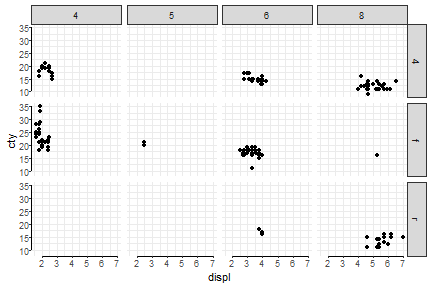The above example is re-created below with both left- and bottom-axis lines repeated.

library(lemon)
p + facet_rep_grid(drv ~ cyl) + coord_capped_cart(bottom='both', left='both') +
theme_bw() + theme(panel.border=element_blank(), axis.line=element_line())
## Coordinate system already present. Adding new coordinate system, which will replace the existing one.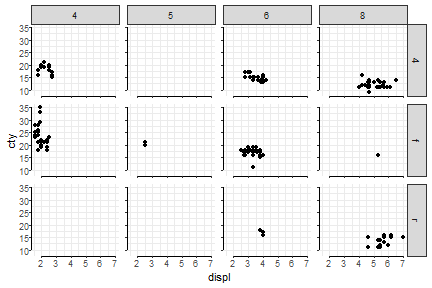Keeping (some) labels

In the following example, we change the facet from a grid to being wrapped on the interaction of drv and cyl, and add free scaling on y-axis. facet_wrap would normally print the y-axis tick labels for each panel, but still ignores the x-axis.

p + facet_wrap(~ interaction(cyl, drv), scales='free_y')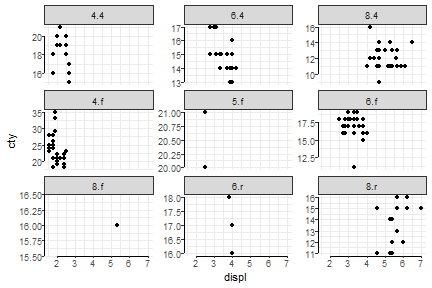A work around by keeping both axes free scale, and fixing the x-axis with either scale_x_continuous or limits in cord_*, but the same x-axis tick labels are repeated. And this is a bit tedious.

p + facet_wrap(~ interaction(cyl, drv), scales='free') +
coord_capped_cart(bottom='both', left='both', xlim=c(2,7))
## Coordinate system already present. Adding new coordinate system, which will replace the existing one.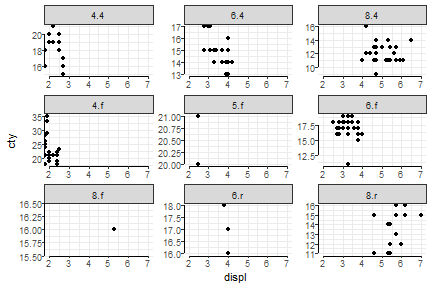We can specify which labels to keep with facet_rep_wrap. Default is repeat.tick.labels=FALSE when scales='fixed' which removes tick labels on all axes (shown in earlier figure). When using free scales on facet_rep_wrap, the appropiate labels are drawn.

p + facet_rep_wrap(~ interaction(cyl, drv), scales='free_y', repeat.tick.labels = 'left')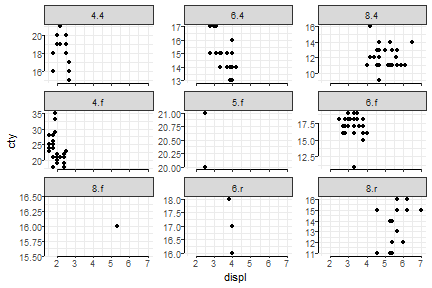The argument repeat.tick.labels accepts a range of arguments: top,right,bottom, and left, any combination of these are accepted, as well as TRUE and FALSE which are interpreted as all or none, and all and none.

Examples

There are many posibilities. Examples given below (but not executed), and they might not be pretty.

p + facet_rep_wrap(~ interaction(cyl, drv), scales='free_y', repeat.tick.labels = 'all')
p + facet_rep_wrap(~ interaction(cyl, drv), scales='free_y', repeat.tick.labels = 'bottom')
p + facet_rep_wrap(~ interaction(cyl, drv), scales='free_y', repeat.tick.labels = 'left')
p + scale_x_continuous(sec.axis = dup_axis()) +
facet_rep_wrap(~ interaction(cyl, drv), scales='free_y', repeat.tick.labels = 'all')
p + scale_x_continuous(sec.axis = dup_axis()) +
facet_rep_wrap(~ interaction(cyl, drv), scales='free_y', repeat.tick.labels = c('top','left'))